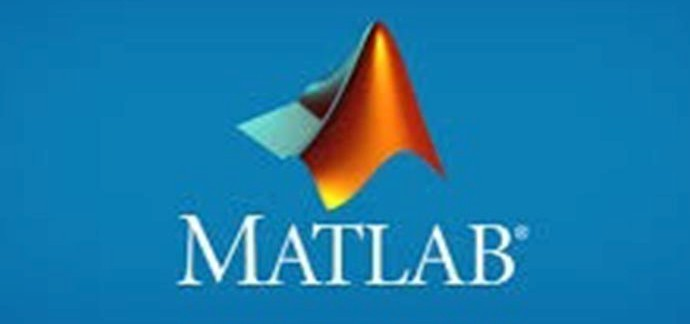• (+91) - 991 814 1007
• info@cosmofoundation.com
MATLAB Basic and Projectdescription

Mat Lab is the programming language for technical computing. It amalgamates visualizing, programming and computation to solve mathematical problems. It assists in matrix and vector formulations. Mat Lab is the tool used for high productivity development and analysis. The tool allows the user to learn and apply specialized technology. The toolboxes are available in the area which includes control system, neural networks, signal processing, wavelets and many others. We are the top best MatLab training institutes in Lucknow.

MATLAB Training
• Providing training with working professional
• Practicals class with real time scenarios
• Lab facilities and real time projects
• Mock interviews
• Job support
Course Overview
Introduction To Basic MATLAB Concepts
• Basic Reading and Writing Data from a File
Introduction To Data Storage And Manipulation
• Data Types and Operations on Point Values.Boolean and Rational
• Strings
• Portable Functions
• Complex Numbers
Arrays And Matrices
• What is an array
• Introduction to array operations
• Vectors and basic vector operations.Structure Arrays
• Cell Arrays
• Sparse Matrices
Introduction To M File Programming
• Scripts
• The Input Function.Control Flow
• Loops and Branches.Error Messages
• MATLAB Caveats.Debugging M Files
Introduction To Mathematical Manipulations
• Linear Algebra
• Simple matrix manipulation
• More complicated matrix operations.Differential Equation
• Ordinary Differential Equations.Partial Differential Equations